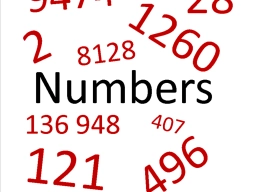# Necessary 81554

It is necessary to determine the number whose double is reduced by 15 equals the number 5.

x =  10

### Step-by-step explanation:

2·x-15=5

2x = 20

x = 20/2 = 10

x = 10

Our simple equation calculator calculates it.Did you find an error or inaccuracy? Feel free to write us. Thank you!

Tips for related online calculators
Do you have a linear equation or system of equations and looking for its solution? Or do you have a quadratic equation?